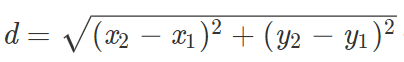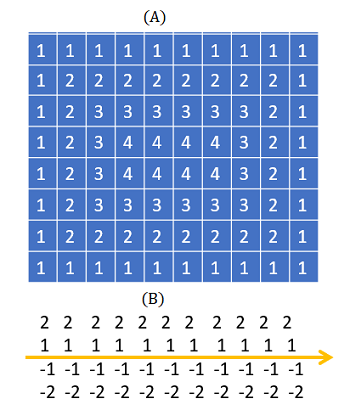# Distance Function: Simple Definition, Examples

## What is a Distance Function?

A distance function measures the distance between two points in a set. For example, you might want to find the distance between two points on a line. A distance function can also apply to other metric spaces.

A metric space is a collection of objects. They can be defined on the Euclidean plane, on functions, matrices, sets of points and many more objects .

## Distance Formula (Euclidean Plane)

For a distance on the Euclidean plane, the distance formula is an application of the Pythagorean theorem:To find the shortest distance between two points, just plug in your two (x, y) values into the formula. For example, let’s say your points were (1, 2) and (9, 4), which means:

• x1 = 1
• x2 = 9
• y1 = 2
• y2 = 4

Plugging these into the formula, we get:Solving, we get the solution: 8.25.

## Distance Function in Shape Analysis & Machine Learning

In machine learning and data analysis, a distance function gives the distance between elements of a set; A distance of zero indicates the elements are equivalent. Methods like classification, clustering, and retrieval require distance metrics to define distances. 

Distance functions are sometimes called a metrics, but there is a difference between a distance function and a distance metric. A distance metric satisfies non-negativity, symmetry and triangle inequality. A distance function doesn’t have to satisfy these properties, so you can think of it as being a more general term .Distance functions in shape analysis show you how far away a point is from an edge (A) or a line (B).

In shape analysis, each pixel of a shape is labeled according to how far a pixel is from the background. A pixel 1 unit away from the background (i.e. and edge of an image) is labeled 1. A pixel 2 units away is labeled 2, and so on. These distances can be all positive, or signed ±.

## References

 Hartenstine, D. (2004). Distance and Metric Spaces. Retrieved April 8, 2021 from: https://www.math.utah.edu/mathcircle/notes/distance.pdf
 Brown, E. Dis-Function: Learning Distance Functions Interactively. Retrieved April 8, 2021 from: http://valt.cs.tufts.edu › brown2012disfunctionPDF
 Xin Sui. Learning Distance Functions. Retrieved April 8, 2021 from: https://www.cs.utexas.edu/~grauman/courses/spring2008/slides/Learning_distance_functions.pdf

CITE THIS AS:
Stephanie Glen. "Distance Function: Simple Definition, Examples" From StatisticsHowTo.com: Elementary Statistics for the rest of us! https://www.statisticshowto.com/distance-function/